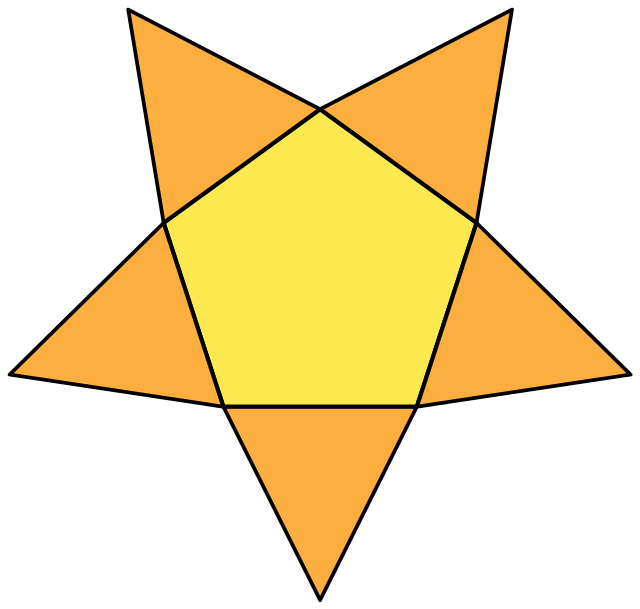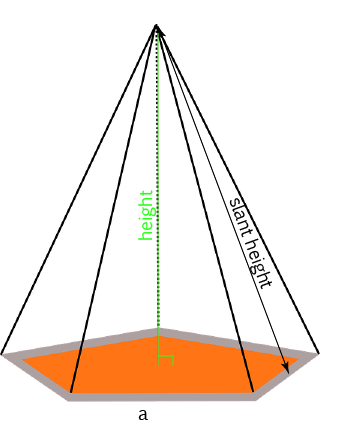# Pentagonal PyramidA pentagonal pyramid is a polyhedron (flat-sided three-dimensional figure), which has a pentagon as its base, and five faces, shaped like triangles, that meet at a point, called the apex.

Pentagonal pyramids have six faces, six vertices and ten edges.## Building a Pentagonal Pyramid

You can make a pentagonal pyramid yourself by using a template like the following net (template), cutting it out along the outside lines, folding it along the inside lines and taping it together along the edges.## Finding the Surface Area and Volume of a Pentagonal Pyramid

### Finding the Surface Area of a Pentagonal PyramidWe can split the surface area of a pentagonal pyramid up into two parts:

1. The Area of the Base.
2. The Area of the Slanted Sides

When the slanted sides are all the same, the surface area of the pentagonal pyramid is given by the formula:

$\text{Surface Area} = (\text{Base Area}) + \dfrac{1}{2} (\text{Perimeter})(\text{Slant Height}).$

### Finding the Volume of a Pentagonal Pyramid

Like any pyramid, the volume of the pentagonal pyramid is equal to $\dfrac{1}{3}$ the volume of the pentagonal prism with the same height and base. So,

$\text{Volume} = \dfrac{1}{3} (\text{Base Area})(\text{Height}).$

### Description

There are several lessons related to 3D geometry such as

1. Euler's formula
2. Vertices, Edges and Faces
3. Volumes of 3D shapes
4. etc

Even though we've titled this lesson series to be more inclined for Year 10 or higher students, however, these lessons can be read and utilized by lower grades students.

### Prerequisites

Understanding of 3D shapes

### Audience

Year 10 or higher, but suitable for Year 8+ students

### Learning Objectives

Get to know 3D Geometry

Author: Subject Coach
You must be logged in as Student to ask a Question.